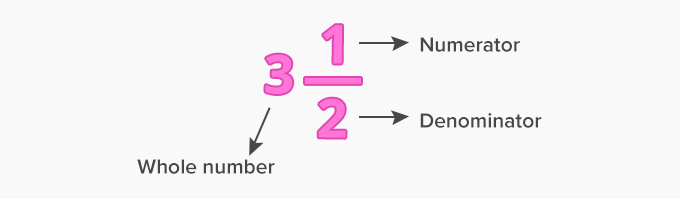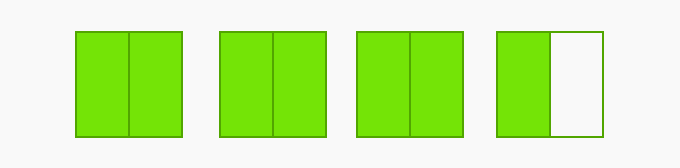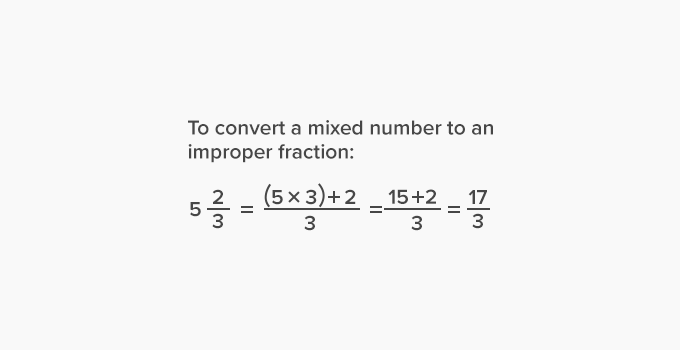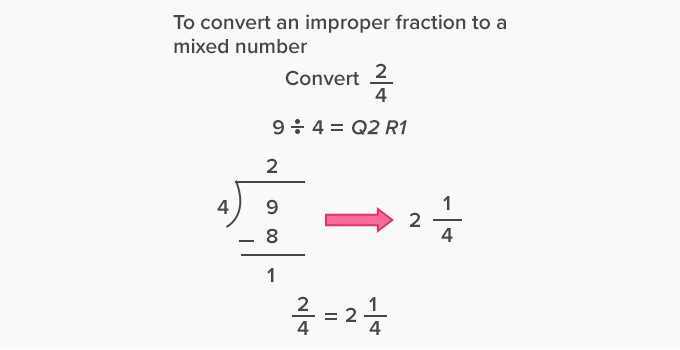# Multiplying Mixed Numbers - Definition with Examples

The Complete K-5 Math Learning Program Built for Your Child

• 30 Million Kids

Loved by kids and parent worldwide

• 50,000 Schools

Trusted by teachers across schools

• Comprehensive Curriculum

Aligned to Common Core

## Multiplying Mixed Numbers

A mixed number has a whole number and a fractional part. The fractional part consists of a numerator and a denominator.Pictorially this can be shown as:Here, we divide each whole into 2 equal parts. So, the denominator of the fraction is 2. There are 7 shaded parts and the fraction represented is  7 2 .

There are 3 whole parts and one-half part. Thus, the fraction represented is  312.Multiplying a mixed number by a whole number:

Step 1: Convert the mixed number into an improper fraction.

Step 2: Rewrite the whole number as a fraction with denominator 1.

Step 3: Multiply two fractions by multiplying the numerators and denominators separately.

Example 1:  212 x 2

First, convert 212 into an improper fraction.

212=(2x2) + 12 = 52

Now, the whole number 2 can be written as a fraction with denominator 1.

212 x 2= 52 x 21  = 5 x 1 1 x 1 = 51= 5

Example 2: 435 x 6

First, convert 435into an improper fraction.

435=(4 x 5)+ 35 = 235

Now, the whole number 6 can be written as a fraction with denominator 1.

435x 6 = 236123 x 65 x 1 = 1381

Now, convert the improper fraction into a mixed number.

138÷5 = Q27 R3

138 = 2735

Thus, 4  x 6= 2735.

Multiplying two mixed numbers:

Step 1: Convert the mixed numbers into improper fractions.

Step 2: Multiply the two fractions by multiplying the numerators and denominators separately.

Example 3: 112 x213.

First, convert 112 and213into improper fractions.

112 = (1 x 2) + 12 = 32

213 = (2 x 3) + 13 =73

Now, multiply the two improper fractions.

112 = 213 =3273

Cancel the common factors and multiply the numerators and denominators separately.

3 2 x 7 3 = 1x7 2x1 = 7 2

Now, convert the improper fraction into a mixed number.

7 ÷ 2 = Q3 R1

72 = 312

Thus,  112 = 213= 312

Example 4: 323= 525

First, convert 323and 525into improper fractions.

323=(3 x 3) + 23 = 113

525=(5 x 5) + 25 = 275

Now, multiply the two improper fractions.

32= 5 25 = 11 3 x  27
5

Cancel the common factors and multiply the numerators and denominators separately.

11 3 x 27 5 = 11
1
x 5 =  11x 9 5 = 995

Now, convert the improper fraction into a mixed number.

99 ÷ 5 = Q19 R4

99= 1945

Thus,

23 x 5 25 = 195

 Fun facts: Multiplying a mixed number by a whole number is performing the repeated addition of the mixed number as many times as the whole number. For example, 11 2× 3, is adding 11 2  three times as:

112x 3 =112 + 112 + 112 = 1 + 1 + 1 +1 2 + 1 2 + 1 2 = 3 + 3 2  = 62 + 32 = 92= 412

Won Numerous Awards & Honors